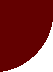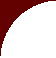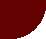Adjusting for Feed Dry Matter LevelFARMLLC home

Milk: Liters to Pounds

 HOW TO ADJUST AS FED AMOUNT FOR CHANGES IN DRY MATTER Karen L. Jacobsen, BS, DVM, MS Farm Animal Resources & Management (F.A.R.M.), LLC Example: You want to feed 16 lb of silage dry matter (DM) per cow per day. You discover that the silage is 30% DM. To calculate how much silage to feed the cows, use the following equation, where X is the unknown amount of silage As Fed. (You are saying that 16 lb is 30% of X amount.) 16 = .30 X 16/.30 = X X= 53.33 lb silage/cow/day Thus, you need to feed 53.33 lb silage cow/day. The "Rule of Thumb" is: Divide the desired pounds of dry matter by the percent dry matter expressed as a decimal.